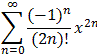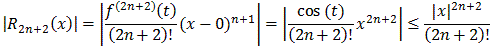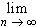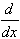#Interactive Real Analysis

Next | Previous | Glossary | Map

## 8.4. Taylor Series

### Proposition 8.4.12: Taylor Series for the Cosine Function

cos(x) = 1 - 1/2! x2 + 1/4! x4 - 1/6! x6 + ... =for all x

Taking derivatives at c=0 we quickly see that all odd derivatives at c=0 are zero while the even ones are plus or minus one. Thus:

cos(x) = 1 - 1/2! x2 + 1/4! x4 - ... + (-1)n/(2n)! x2n + R2n+2(x)

We need to show that the remainder R2n+2 goes to zero as n goes to infinity. Let's use the Lagrange remainder with c=0:But for a fixed x we have already shown before that for any fixed number x|x|n/n! = 0

which finishes the proof.### Fun Facts:

1. cos(0) = 1
2. cos is an even function, i.e. cos(-x) = cos(x)
3. cos(x -/2) = -sin(x)
4.cos(x) = - sin(x)

The fun facts are all easy and are left as exercises. For the third fact you might start with f(x)=sin(x) and develop that in a series around c =/2.

Next | Previous | Glossary | Map